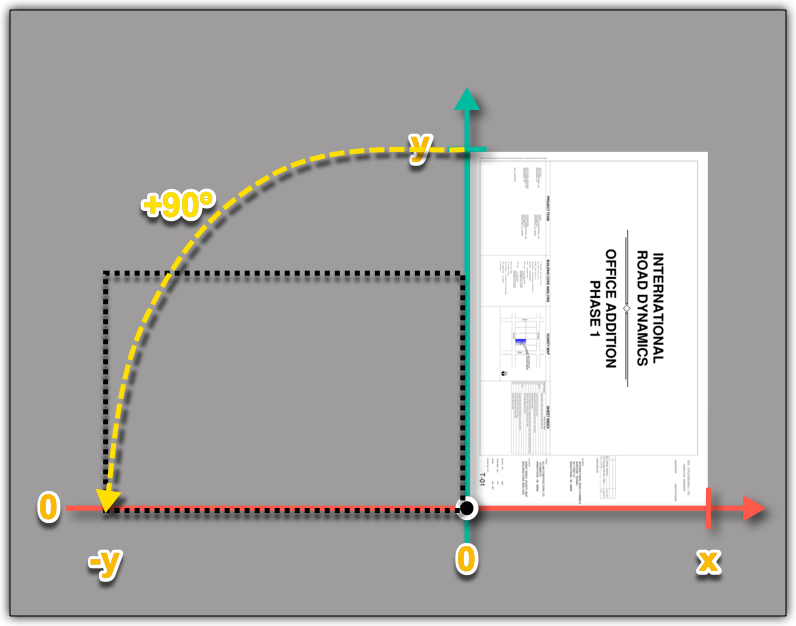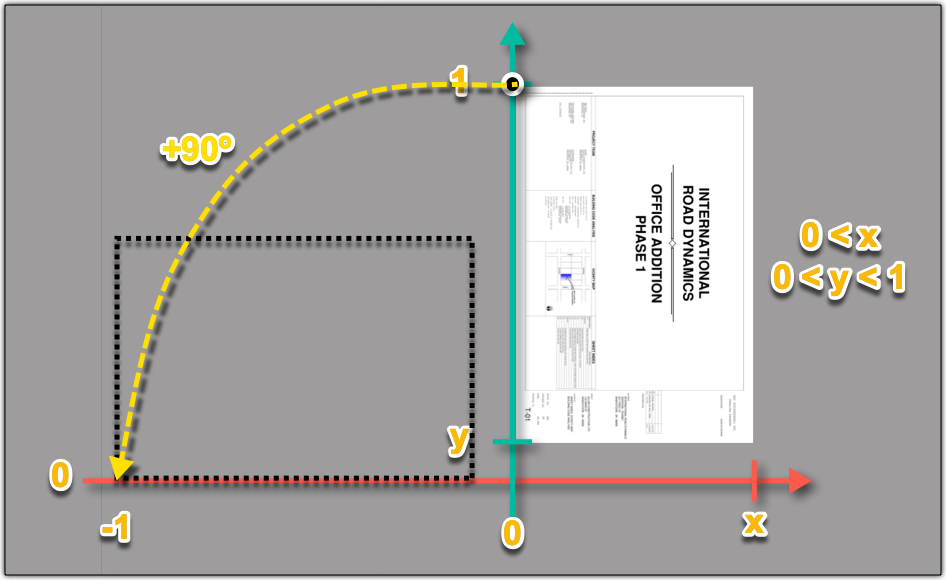December 17, 2020

# Rotate sheets in the ViewerIf you just want to rotate a sheet in the Viewer, that's quite simple. You can set the rotation before or after loading the sheet.

Before:

``````var mx = new THREE.Matrix4();
mx.makeRotationZ(90 * Math.PI / 180);

After:

``````viewer.loadModel("TestSheet.pdf", {page: 1}, (model) => {
var mx = new THREE.Matrix4();
mx.makeRotationZ(90 * Math.PI / 180);
model.setPlacementTransform(mx);
});``````

It's a bit more complicated if you want to load multiple sheets (one vertical, the other horizontal), rotate one of them, and also align them - e.g. in order to compare them, see PixelCompare extension

Let's say we want to make a vertical page horizontal by rotating it +90º (PI/2 radian)In case of PdfLoader, the bottom left corner of the page is always at the origin (0, 0) and its width will extend x along the X axis and its height will reach y along the Y axis - see picture on top.
The rotation always happens around the origin, but in the matrix we can also store values for translation (moving to final position)
After rotation, the new bottom left corner will be at (-y, 0), so we need to add (y, 0) translation to the matrix in order to move that corner back to the expected (0, 0) position

``````viewer.loadModel("TestSheet.pdf", {}, (model) => {
var bb = model.getBoundingBox();
var mx = new THREE.Matrix4();
mx.makeRotationZ(90 * Math.PI / 180);
// translation along X axis
mx.elements = bb.max.y;
model.setPlacementTransform(mx);
});``````In case of LeafletLoader, the top left corner of the page is always at (0, 1) and its width will extend x along the X axis and its height will go from y to 1 along the Y axis
After rotation, the new top left corner will be at (-1, x), so we need to add (1, 1-x) translation to the matrix in order to move that corner back to the expected (0, 1) position

``````viewer.loadModel("TestSheet.pdf", {}, (model) => {
var bb = model.getBoundingBox();
var mx = new THREE.Matrix4();
mx.makeRotationZ(90 * Math.PI / 180);
// translation along X and Y axes
mx.elements = 1;
mx.elements = 1 - bb.max.x;
model.setPlacementTransform(mx);
});``````